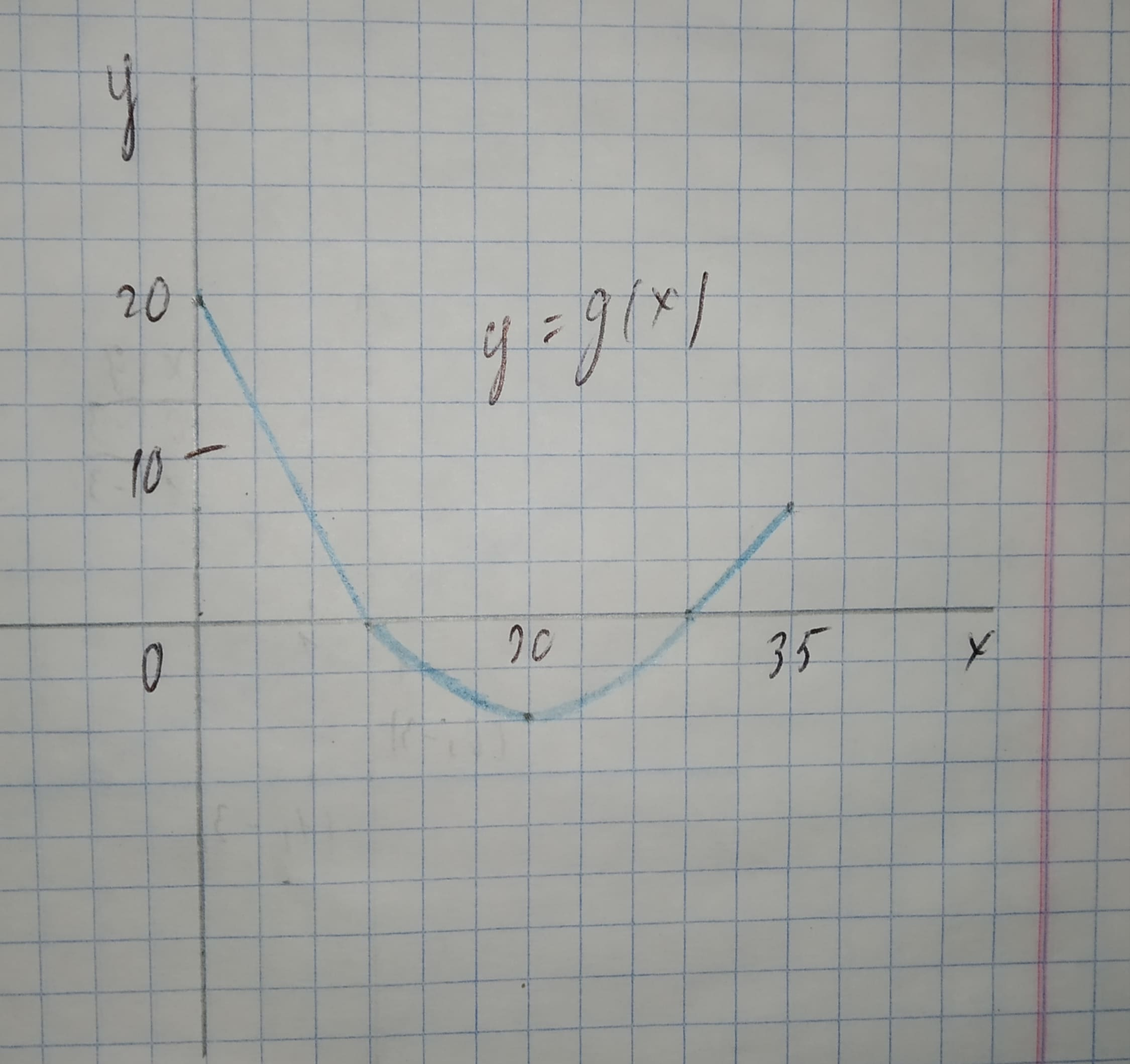# The graph of g consists of two straight lines and a semicircle. Use it to eveluate the integral.12210203861.jpgint_0^35 g(x)dxArmorikam 2021-02-19 Answered

The graph of g consists of two straight lines and a semicircle. Use it to eveluate the integral.${\int }_{0}^{35}g\left(x\right)dx$

You can still ask an expert for help

• Questions are typically answered in as fast as 30 minutes

Solve your problem for the price of one coffee

• Math expert for every subject
• Pay only if we can solve itMargot Mill
The integral ${\int }_{0}^{35}g\left(x\right)dx$ is the area between the graph of g and the horizontal x-axis on the interval $0\le x\le 35$. We separate this integral into the parts of (a) and (b) along with
${\int }_{30}^{35}g\left(x\right)dx$.
${\int }_{30}^{35}g\left(x\right)dx$ represents the area that forms a triangle with base b = 5 and height h = 5.
The area of a triangle is the product of the base b and the height h, divided by 2.
${\int }_{30}^{35}g\left(x\right)dx=\frac{b\cdot h}{2}$
$=\frac{5\cdot 5}{2}$
$=\frac{25}{2}$
=12.5